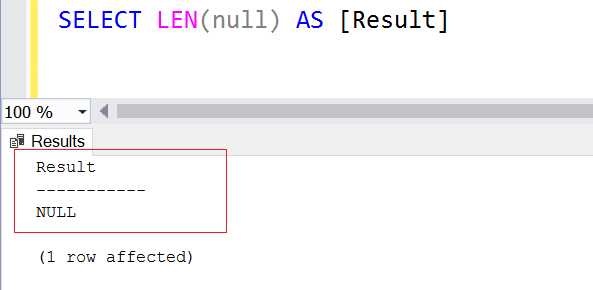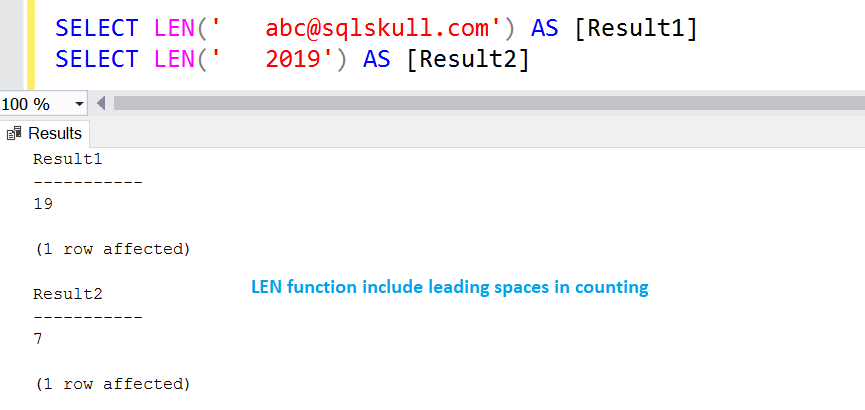Home » SQL LEN()

# SQL LEN()SQL LEN() function is used to count the number or length of character in given string. It returns integer value always and null for empty string.

It does not count the trailing spaces of string.

SYNTAX

`LEN(string)`

Lets look at an example of LEN() function in SQL.

#### Using LEN function to count the length of string

```SELECT LEN('abc@sqlskull.com') AS [Result1]
SELECT LEN(2019) AS [Result2]```As you can see first SQL statement counts the length of mailid and second statement counts the length of year.

#### LEN function with empty value

Len Function returns 0 for empty value as shown in below screenshot.

SELECT LEN(”) AS [Result]#### LEN function with null value

If input string is null then it LEN function returns null as shown below.

`SELECT LEN(null) AS [Result]`#### LEN function returns an error when Input string is not provided

If input string are not specified then it returns an error message as you can see in below screenshot.

`SELECT LEN() AS [Result]`#### LEN function with string contains trailing spaces

If string contains trailing spaces then len() function will not include them in counts.

Following statement uses LEN function and counts the len of string excluding trailing spaces.

```SELECT LEN('abc@sqlskull.com   ') AS [Result1]
SELECT LEN('2019   ') AS [Result2]```

String ‘abc@sqlskull.com   ‘ contains three trailing spaces and string ‘2019   ‘ also contains three trailing spaces.As you see, a mail id string contains three trailing spaces and year string also contains three trailing spaces but LEN function exclude the trailing spaces from counting and returns Length of string.

#### LEN function with string contains leading spaces

If string contains leading spaces then len() function also counts leading spaces.

Following statement uses LEN function and counts the len of string including leading spaces.

```SELECT LEN('   abc@sqlskull.com') AS [Result1]
SELECT LEN('   2019 ') AS [Result2]```

String ‘   abc@sqlskull.com’ contains three leading spaces and string ‘   2019’ also contains three leading spaces.As you can see LEN function also counts the leading spaces in string. The actual length of mail id string  is 16 and there are 3 leading space so the total length of string is 19 returned by LEN function.

In similar way the Len of Year string is 4, and it contains 3 leading spaces so the total length of string becomes 7.

#### Using LEN Function counts the len of values in column.

Here we have a table named EmpDpt, following are the table scripts

``` CREATE TABLE dbo.EmpDpt
(Id INT,
Name VARCHAR(50),
Department VARCHAR(50)
)

INSERT INTO dbo.EmpDpt
(Id, Name, Department)
VALUES
(1, 'Jack Hanery', 'IT'),
(2, 'Joshep Mac', 'HR'),
(3, 'Rozer Jr', 'IT'),
(4, 'Mustafha Md.', 'Finance')```

Lets count the length of employee Name.

```SELECT Id, Name, LEN(NAME) AS NAME_LEN
FROM dbo.EmpDpt```As you can see, the length of employee name is in column NAME_LEN.